# SI UnitsPage 1

#### WATCH ALL SLIDES

Slide 1## The Système International

SI Units

Slide 2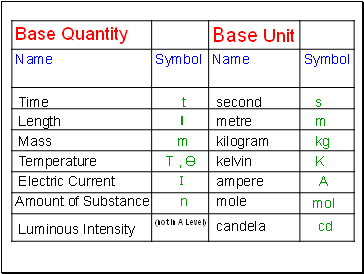Time

Length

Mass

Temperature

Electric Current

Amount of Substance

Luminous Intensity

t

l

m

T , Ө

I

n

(not in A Level)

second

metre

kilogram

kelvin

ampere

mole

candela

s

m

kg

K

A

mol

cd

Slide 3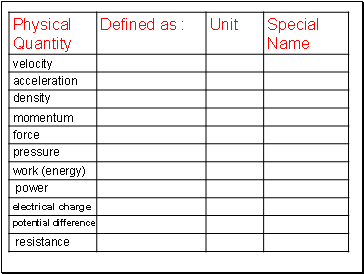density

momentum

force

pressure

work (energy)

power

electrical charge

potential difference

resistance

Slide 4## Homogenous Equations

Ep = m g h

=

Nm = kg kgms-2kg-1 m

kgms-2 m = kgm2s-2

kgm2s-2 = kgm2s-2

 This equation is homogenous

J

kg

Nkg-1

m

Slide 5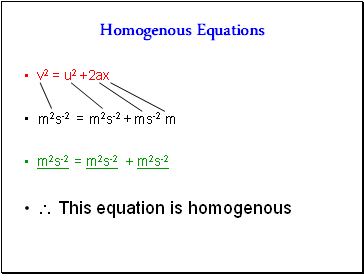Homogenous Equations

v2 = u2 +2ax

= +

m2s-2 = m2s-2 + m2s-2

 This equation is homogenous

m2s-2

m2s-2

ms-2

m

Slide 6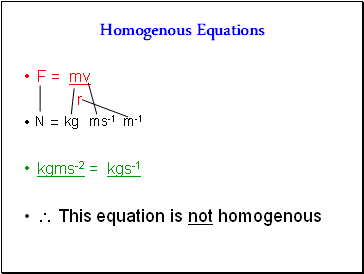Homogenous Equations

F = mv

r

=

kgms-2 = kgs-1

 This equation is not homogenous

N

kg

ms-1

m-1

Slide 7Homogenous Equations

What is missing? kgms-2 = kgs-1

F = mv2

r

ms-1 =

v on rhs

Slide 8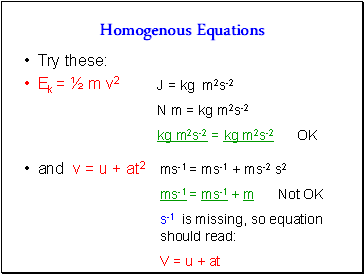Homogenous Equations

Try these:

Ek = ½ m v2

and v = u + at2

J = kg m2s-2

N m = kg m2s-2

kg m2s-2 = kg m2s-2 OK

ms-1 = ms-1 + ms-2 s2

ms-1 = ms-1 + m Not OK

s-1 is missing, so equation should read:

V = u + at

Slide 9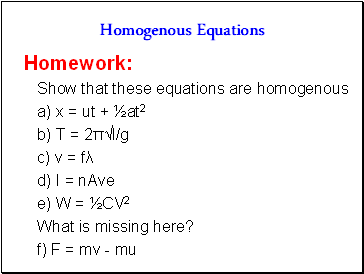Homogenous Equations

Homework:

Show that these equations are homogenous

a) x = ut + ½at2

b) T = 2π√l/g

c) v = fλ

d) I = nAve

e) W = ½CV2

What is missing here?

f) F = mv - mu